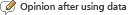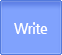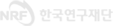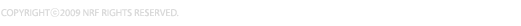Search
Search

#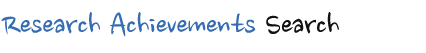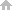HOME > Search by Achievements Type > Reports View

#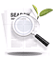Reports Detailed Information

Integral Points and Rank of Some Elliptic Curves
Reports NRF is supported by Research Projects( Integral Points and Rank of Some Elliptic Curves | 2004 Year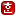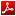| 김선미(전북대학교)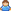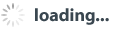) data is submitted to the NRF Project Results
Researcher who has been awarded a research grant by Humanities and Social Studies Support Program of NRF has to submit an end product within 6 months(* depend on the form of business)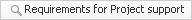• Researchers have entered the information directly to the NRF of Korea research support system
 Project Number C00001 Year(selected) 2004 Year the present condition of Project 종료 State of proposition 재단승인 Completion Date 2008년 09월 19일 Year type 결과보고 Year(final report) 2008년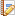Research Summary
•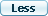Korean
• 본 논문에서는 특별한 타원곡선에서의 integral points와 그 rank에 관해 연구하였다. 제 2장에서는 타원곡선을 연구하기 위해 필요한 개념과 정리들: Projective space, Affine space, 타원곡선 그리고 타원곡선에서 정의된 연산자에 대해서 소개하였다.

제 3장에서는 Valuations 과 Absolute values를 정의하였다.

끝으로, 제 4장에서는 weak Mordell-Weil, Mordell-Weil, Lutz-
Nagell 그리고, Mazur 정리등 타원곡선의 rank와 torsion subgroup를 구하는데 도움이 되는 정리들을 소개하였다.

그리고, 이 정리들을 이용해 theorem 4.18, 4.19에서는 특별한 조건의 와 에 관한 타원곡선 E_D와 E_B의 torsion subgroup를 구할 것이다.

Theorem 4.20에서는 타원곡선의 rank에 관한 upper bound를 증명 할 것이다. 이 증명을 타원곡선 E_D와 E_B에 적용하여 이 두 타원곡선의 rank에 관한 upper bound를 구할 것이다. 그리고 타원곡선 y^2 = x^3 + 4 x 의 rank가 이 됨을 보이고, 타원곡선 y^2 = x^3 + 4 x와 타원곡선 y^2 = x^3 + 4 m^4 x 이 isomorphic함을 이용해 타원곡선 y^2 = x^3 + 4 m^4 x의 rank가 이 됨을 보일 것이다.
또한, Corollary 4.27에서는 특별한 조건을 만족하는 에 관한 타원곡선 (,)의 rank에 관한 upper bound를 구하였다.
감 사 의 글
•English
• This paper is a study of the integral points and the rank on some elliptic curve.

In the second chapter, we introduce necessary notions and facts which works on the elliptic curves
: projective space, elliptic curves, and the addition operation which works on the points of the curve.

In the third chapter, we will deals with valuations and absolute values.

In the fourth chapter, we will finally research some theorems which help us explicitly analyze the torsion subgroup, $E_{tors} ( {\Bbb Q} )$, and the rank of the elliptic curve ; the weak Mordell-Weil, Mordell-Weil, Lutz-Nagell and Mazur theorems.

And, we will use those theorems to construct the torsion group $E_{tors} ( {\Bbb Q} )$ for the elliptic curves $E_D$ and $E_B$ is given by special conditions $D$ and $B$.

We will demonstrate the upper bound of the rank in the theorem $4.20.$ Using this theorem, we will calculate the upper bound of the rank on the elliptic curves $E_D$ and $E_B$, which are given by special conditions $D$ and $B$.
And we will calculate that the rank of the elliptic curve $y^2 = x^3 + 4 x$. If we use the fact that the elliptic curve $y^2 = x^3 + 4 x$ is isomorphic to the elliptic curve $y^2 = x^3 + 4 m^4 x$, then can show that the rank of the elliptic curve $y^2 = x^3 + 4 m^4 x$ is zero.

Also, we will calculate the upper bound of the rank on the elliptic curve $E_{\alpha}$ with special conditions for $\alpha$.Research result report
•Abstract
• This paper is a study of the integral points and the rank on some elliptic curve.

In the second chapter, we introduce necessary notions and facts which works on the elliptic curves
: projective space, elliptic curves, and the addition operation which works on the points of the curve.

In the third chapter, we will deals with valuations and absolute values.

In the fourth chapter, we will finally research some theorems which help us explicitly analyze the torsion subgroup, $E_{tors} ( {\Bbb Q} )$, and the rank of the elliptic curve ; the weak Mordell-Weil, Mordell-Weil, Lutz-Nagell and Mazur theorems.

And, we will use those theorems to construct the torsion group $E_{tors} ( {\Bbb Q} )$ for the elliptic curves $E_D$ and $E_B$ is given by special conditions $D$ and $B$.

We will demonstrate the upper bound of the rank in the theorem $4.20.$ Using this theorem, we will calculate the upper bound of the rank on the elliptic curves $E_D$ and $E_B$, which are given by special conditions $D$ and $B$.
And we will calculate that the rank of the elliptic curve $y^2 = x^3 + 4 x$. If we use the fact that the elliptic curve $y^2 = x^3 + 4 x$ is isomorphic to the elliptic curve $y^2 = x^3 + 4 m^4 x$, then can show that the rank of the elliptic curve $y^2 = x^3 + 4 m^4 x$ is zero.

Also, we will calculate the upper bound of the rank on the elliptic curve $E_{\alpha}$ with special conditions for $\alpha$.
•Research result and Utilization method

• 암호론에서 E({\Bbb Q}) 의 원소를 계산하는 것은 암호를 얼마나 효율적으로 만들 수 있는가라는 문제와 직결되는 문제이다. 타원곡선의 rank에 관한 upper bound를 구함으로써 암호론에서 암호체계를 구현하는데 도움이 되리라 사료 된다.
• Index terms
• elliptic curve, rank
•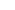List of digital content of this reports• This document, it is necessary to display the original author and you do not have permission